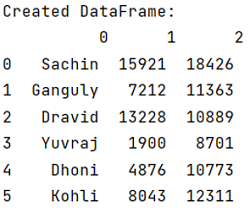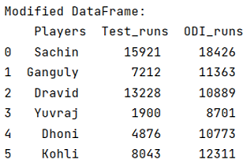Submitted by Pranit Sharma, on May 27, 2022

Pandas is a special tool which allows us to perform complex manipulations of data effectively and efficiently. Inside pandas, we mostly deal with a dataset in the form of DataFrame. DataFrames are 2-dimensional data structure in pandas. DataFrames consists of rows, columns and the data.

Columns are the different fields which contains their particular values when we create a DataFrame. We can perform certain operations on both rows & column values. Each column has specific header/name. We can add a header row by simply assigning a list of names that we want to give as the header in front of DataFrame.columns =

To work with pandas, we need to import pandas package first, below is the syntax:

```import pandas as pd
```

Let us understand with the help of an example.

```# Importing pandas package
import pandas as pd

# Crerating an array
arr1 = ['Sachin', 15921, 18426]
arr2 = ['Ganguly', 7212, 11363]
arr3 = ['Dravid', 13228, 10889]
arr4 = ['Yuvraj', 1900, 8701]
arr5 = ['Dhoni', 4876, 10773]
arr6 = ['Kohli', 8043, 12311]

final_arr = [arr1,arr2,arr3,arr4,arr5,arr6]

# Creating a DataFrame
df = pd.DataFrame(final_arr)

# Display created DataFrame
print("Created DataFrame:\n",df,"\n")
```

Output:```# Adding column headers
df.columns = ['Players','Test_runs','ODI_runs']

# Display modified DataFrame
print("Modified DataFrame:\n",df)
```

Output:Languages: » C » C++ » C++ STL » Java » Data Structure » C#.Net » Android » Kotlin » SQL
Web Technologies: » PHP » Python » JavaScript » CSS » Ajax » Node.js » Web programming/HTML
Solved programs: » C » C++ » DS » Java » C#
Aptitude que. & ans.: » C » C++ » Java » DBMS
Interview que. & ans.: » C » Embedded C » Java » SEO » HR
CS Subjects: » CS Basics » O.S. » Networks » DBMS » Embedded Systems » Cloud Computing
» Machine learning » CS Organizations » Linux » DOS
More: » Articles » Puzzles » News/Updates